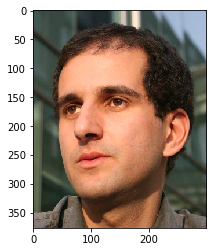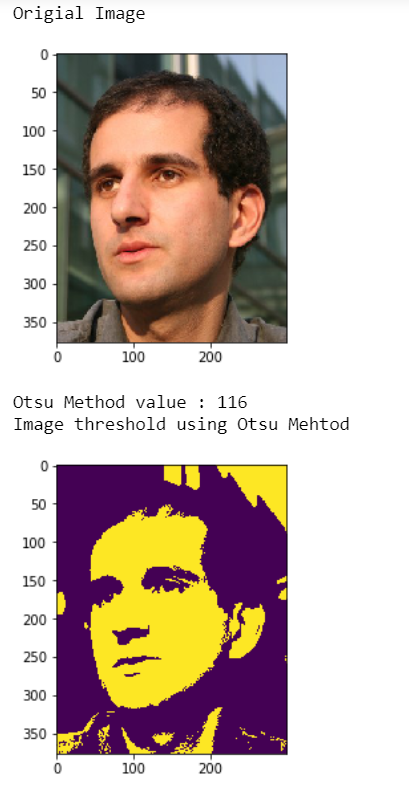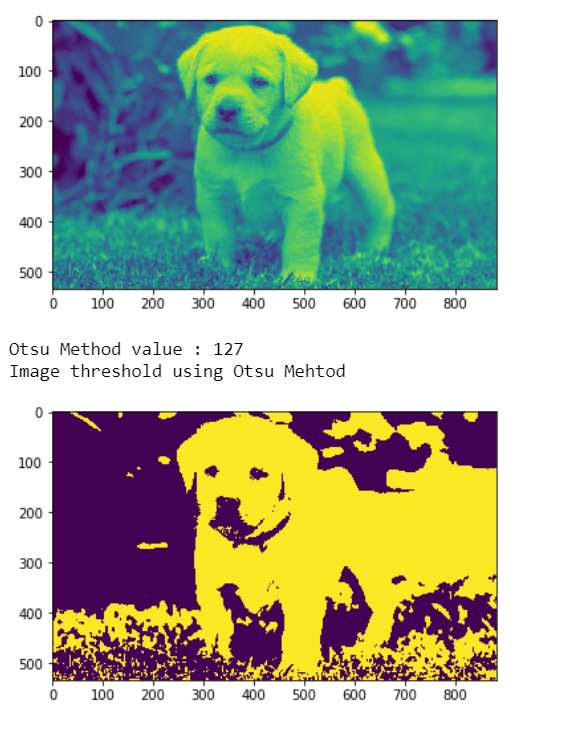# Mahotas – Otsu’s method

In this article we will see how we can implement otsu’s method in mahotas. In computer vision and image processing, Otsu’s method, named after Nobuyuki Otsu, is used to perform automatic image thresholding. In the simplest form, the algorithm returns a single intensity threshold that separate pixels into two classes, foreground and background.

In this tutorial we will use “luispedro” image, below is the command to load it.

`mahotas.demos.load('luispedro')`

Below is the luispedro imageIn order to do this we will use `mahotas.otsu` method

Syntax : mahotas.otsu(image)

Argument : It takes image object as argument

Return : It returns integer

Note : Input image should be filtered or should be loaded as grey

In order to filter the image we will take the image object which is numpy.ndarray and filter it with the help of indexing, below is the command to do this

`image = image[:, :, 0]`

Example 1:

 `# importing required librries ` `import` `mahotas ` `import` `mahotas.demos ` `import` `numpy as np ` `from` `pylab ``import` `imshow, gray, show ` `from` `os ``import` `path ` ` `  `# loading the image ` `photo ``=` `mahotas.demos.load(``'luispedro'``) ` ` `  `# showing original image ` `print``(``"Origial Image"``) ` `imshow(photo) ` `show() ` ` `  `# loading image as grey ` `photo ``=` `mahotas.demos.load(``'luispedro'``, as_grey ``=` `True``) ` ` `  `# converting image type to unit8 ` `# beacuse as_grey returns floating values ` `photo ``=` `photo.astype(np.uint8) ` ` `  `# otsu method ` `T_otsu ``=` `mahotas.otsu(photo) ` ` `  `# printing otsu value ` `print``(``"Otsu Method value : "` `+` `str``(T_otsu)) ` ` `  `print``(``"Image threshold using Otsu Mehtod"``) ` `# showing image ` `# image values should be greater than otsu value ` `imshow(photo > T_otsu) ` `show() `

Output :Example 2:

 `# importing required libraries ` `import` `mahotas ` `import` `numpy as np ` `from` `pylab ``import` `imshow, show ` `import` `os ` ` `  ` `  `# loading iamge ` `img ``=` `mahotas.imread(``'dog_image.png'``) ` `     `  ` `  `# setting filter to the image ` `img ``=` `img[:, :, ``0``] ` ` `  `imshow(img) ` `show() ` ` `  ` `  `# otsu method ` `T_otsu ``=` `mahotas.otsu(img) ` ` `  `# printing otsu value ` `print``(``"Otsu Method value : "` `+` `str``(T_otsu)) ` ` `  `print``(``"Image threshold using Otsu Mehtod"``) ` `# showing image ` `# image values should be greater than otsu value ` `imshow(img > T_otsu) ` `show() `

Output :My Personal Notes arrow_drop_upCheck out this Author's contributed articles.

If you like GeeksforGeeks and would like to contribute, you can also write an article using contribute.geeksforgeeks.org or mail your article to contribute@geeksforgeeks.org. See your article appearing on the GeeksforGeeks main page and help other Geeks.

Please Improve this article if you find anything incorrect by clicking on the "Improve Article" button below.

Article Tags :

1

Please write to us at contribute@geeksforgeeks.org to report any issue with the above content.